# Valence Electrons - What Is This?

By: Henrique Bertulino

9 min

0

10.04.2022Atoms are not connected randomly during molecules' formation but in a certain sequence by special chemical bonds electron shell. Each atom can form not an infinite, but only a certain number of such bonds. In the 19th century, scientists found that atoms of different elements have different abilities to attach other atoms to themselves.

Valence is the number of bonds a particular atom can form with other atoms. The valence electron can absorb and release energy in the form of a photon. Graphical formulas are used to show how atoms are connected in a molecule. They show not only the number of atoms in a molecule but also the sequence of their connection. The graphic formula of the H2O water molecule is written as follows: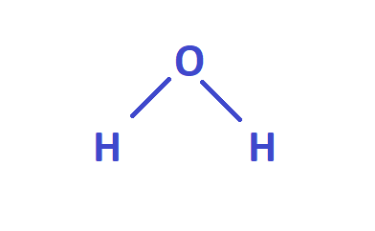From this formula, you can see that the oxygen atom is connected to two hydrogen atoms in the molecule, and the hydrogen atoms are not connected. Each chemical bond in a graphical formula is affected by a trait. The oxygen atom forms two bonds. Therefore, the oxygen valence is two, and the hydrogen atoms from one bond each - the hydrogen valence is one. The highest energy, the more the electron changes. For example, to move to an atom's outermost shell or to escape from the shell, creating ionization.

Note that an atom with a closed shell of valence electrons (corresponding to the electronic configuration for main group elements or transition metals) tends to be chemically inert. Atoms with one or two valence electrons larger than the closed-shell are highly reactive.

## Types of Valence

All elements can be divided into three groups:

• with constant valence;
• with variable valence;
• with zero valences.

The constant valence of chemical elements does not change:

• in alkali metals, fluorine (I);
• in alkaline earth metals, oxygen (II);
• in aluminum (III).

Variable valence is characteristic of the rest of the elements. These elements' atoms contain a d-sublevel, which can accept electrons from the s- and p-sublevels.

The so-called zero valences are inert or noble gases. On the outer energy level, they can contain up to eight electrons. However, these elements are chemically inactive and do not form bonds with other atoms.

Some non-metals can attract additional valence electrons to create a complete shell. There are two ways to do this: an atom can share electrons with a neighboring atom or remove electrons from another atom. For example, this is Halogen. Its electronic configuration looks like this: s2p5. It takes one electron to form a complete shell. To create a covalent bond, one electron of the Halogen and one electron of another atom form a pair.

## How to Determine the Valence of an Element Using the Periodic Table?

The periodic table allows you to determine the basicity of elements quickly. To do this, you need to be able to read the periodic table. The table has eight groups vertically, and periods are located horizontally. If the period consists of two rows, then it is called significant, and if from one - small. Elements are vertically in columns, unevenly distributed in groups. Roman numerals always indicate valence.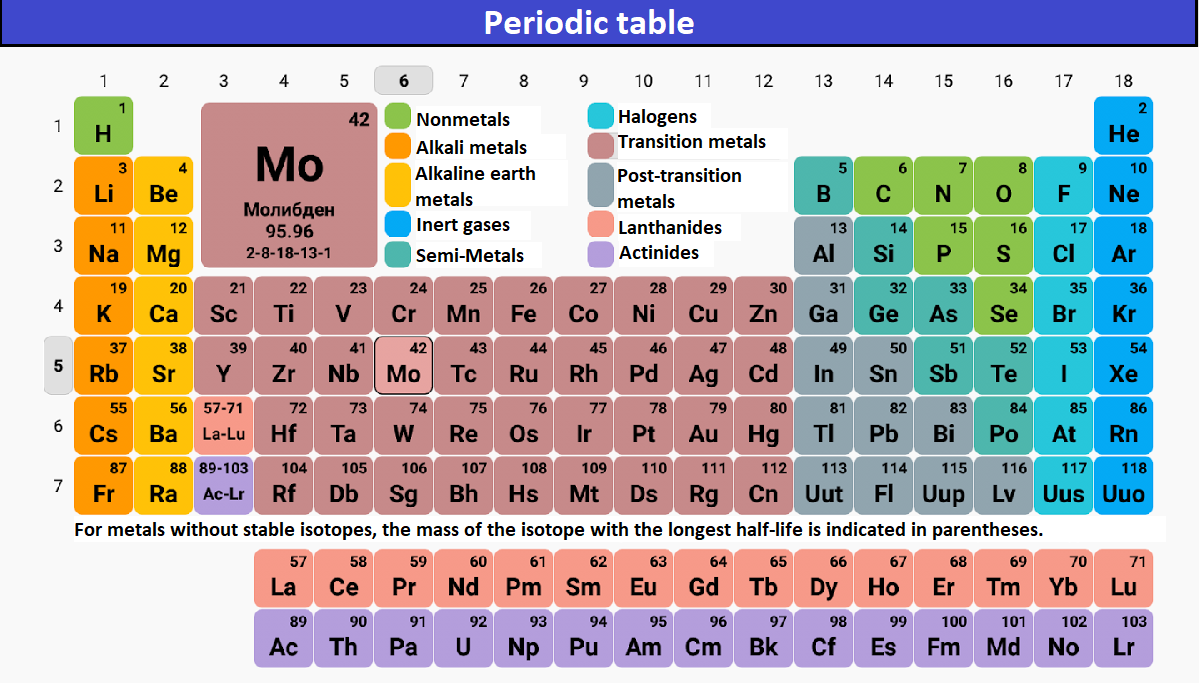To determine the valency, you must follow the universal algorithm:

1. We write down the compound formula.
2. We put down the valence of the component of the compound that we know.
3. We multiply the known value by the number of atoms of the element in the compound.
4. We're looking for the smallest multiple.
5. We check: we multiply the value by the index.

The result should be the exact figure for each component of the compound. Example: Let's take an ammonia compound - NH3. We know that the hydrogen atom has a constant valence and is equal to I. Multiply I by 3 (number of valence electrons) - the smallest multiple - 3. Nitrogen in this formula has an index similar to one. Hence the conclusion: we divide 3 by 1, and we get that for nitrogen, it is equal to IIII. The value for hydrogen and oxygen is always easy to determine.

## How to Find Out Valence From the Valence Table?

The valence of a chemical element can be viewed in a particular table of chemical elements' valence. But such a table is not always given in textbooks. If you do not have such a table at hand, use the method we described above.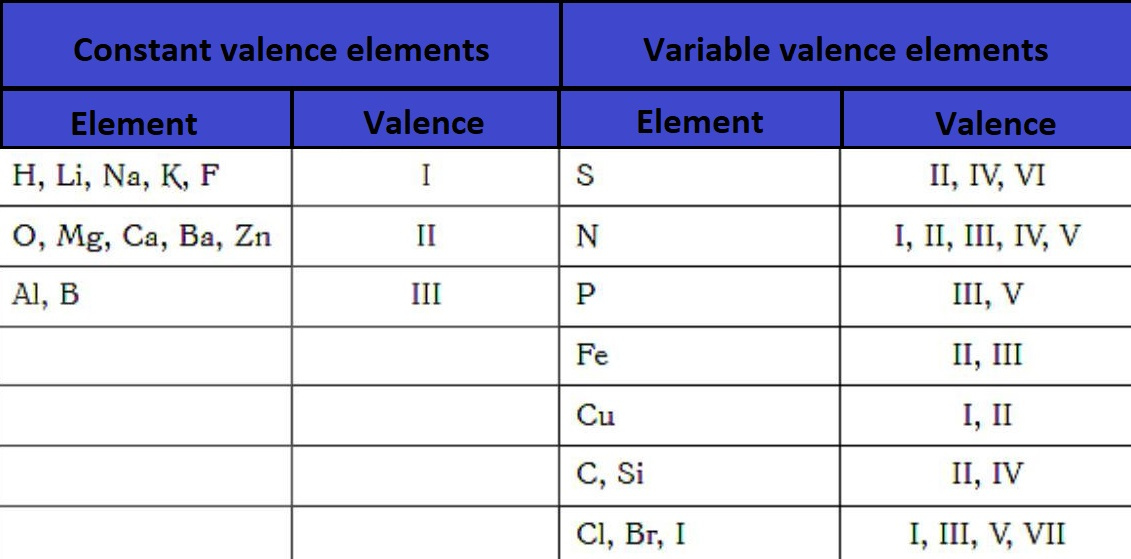Let's take a closer look at the table. The valency of hydrogen is taken as one since a hydrogen atom can form one bond with other atoms. The valence of different elements is expressed by a number that shows how many hydrogen atoms an atom of a given element can attach to itself. For example, the valence of chlorine in a hydrogen chloride molecule is equal to one. Therefore, the formula for hydrogen chloride will look like this: HCl. Since both chlorine and hydrogen have a valency of one, no subscript is used. Both chlorine and hydrogen are monovalent since one hydrogen atom corresponds to one chlorine atom.

## How to Determine Formula Valence?

If you do not have a table of valency in front of your eyes, but there is a formula for a chemical compound, it is possible to determine the formula's valency. Take, for example, the formula for manganese oxide - Mn2O7. The formula looks like this: 2KMnO4 + H2SO4 = K2SO4 + H2O + Mn2O7.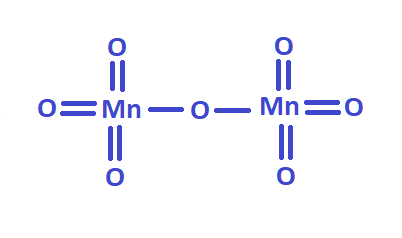As you know, oxygen is bivalent. To determine what valence manganese has, you must multiply the oxygen valence by the number of gas atoms in this compound: 2 ∙ 7 = 14. The resulting number is divided by the number of manganese atoms in the compound. It turns out: 14 : 2 = 7. It turns out that 7 (VII) is the valence of manganese in this chemical reaction.

## Why Do Chemical Elements Have a Certain Valence And Location?

If you mix up the period, group, or position of an element, you will not solve the task. Therefore, you must understand why the elements have such an arrangement and their group, period, depends on.

The arrangement of chemical elements in Mendeleev's periodic table is explained by how electrons fill the available energy levels, or layers, in an atom. For example, noble gases such as neon, xenon, and argon each have 8 electrons in the outer layer - and therefore, they are reluctant to enter into chemical reactions. In most cases, atoms with a filled outer electron layer have the lowest energy state. This is what the octet rules are based on.

The octet rule explains how atoms form ionic elements. Let's take sodium as an example. Its atom has 11 electrons: two in the inner layer, eight in the next, and one in the outer layer. This outer electron is very mobile, so if energy is transferred to the sodium atom, it quickly forms a sodium ion with a single positive charge. It takes ten times more energy to remove an electron from the inner layer, so a sodium ion with a double positive charge is very rare. Likewise, calcium, which has 2 electrons in the outer layer and 8 in the next lower layer, forms an ion, losing 2 electrons. When atoms turn into ions, they become similar in structure to atoms of noble gases.

## Electron Configuration

The electronic configuration records the distribution of electrons in its atoms over the outer shell (outermost shell), inner shell, subshells, and orbitals. The electronic configuration is usually written for atoms in their ground state. The electronic configuration in which one or more electrons are in an excited state is called an exciting configuration.

There is a conventional image of electronic levels and sublevels called an orbital diagram. On such a diagram, orbitals are conventionally depicted by squares and electrons by arrows. If the hands are directed in different directions, this means that the electrons differ from each other by a particular property called the electron spin.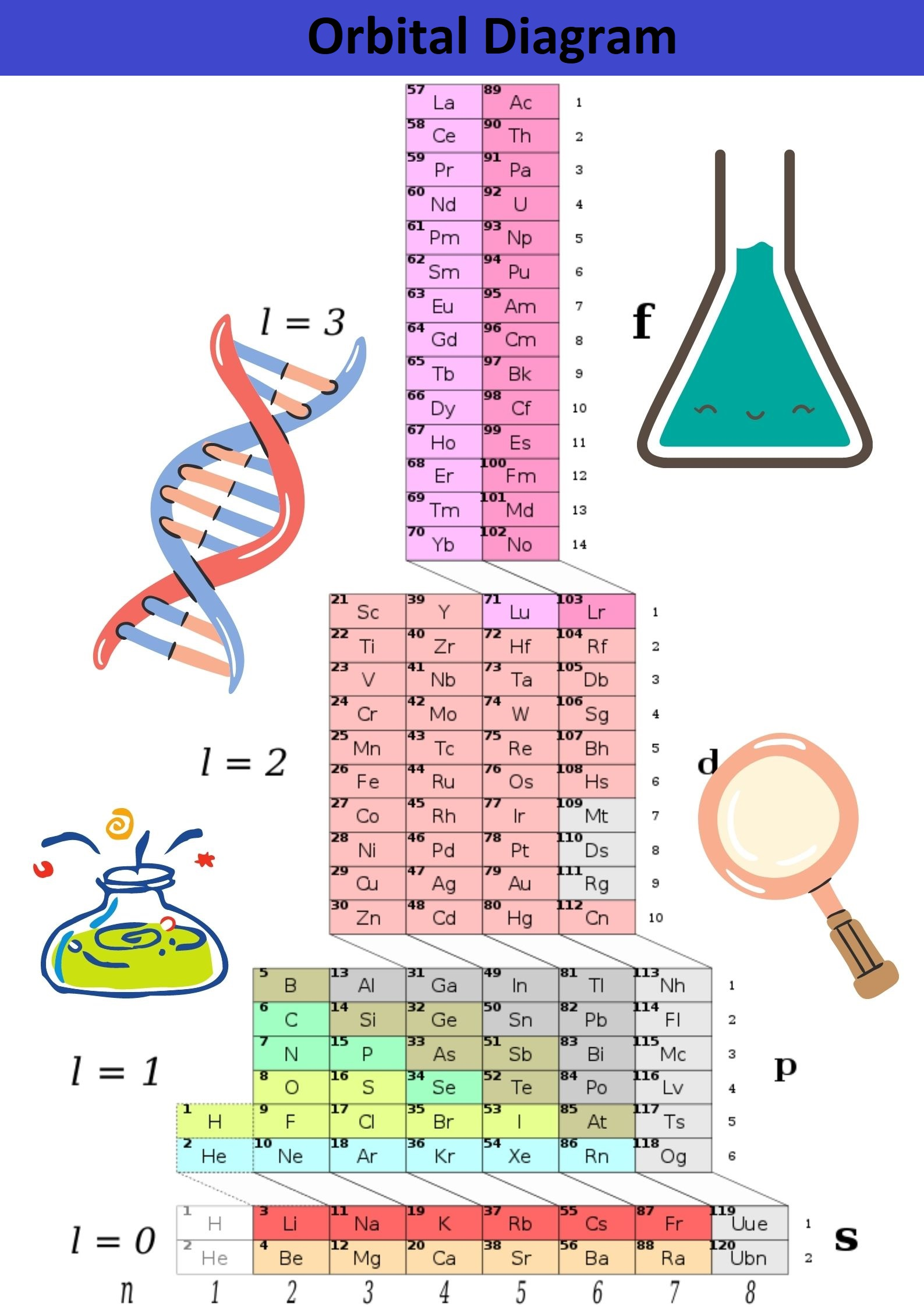### Why Study the Electronic Configuration of Elements in the Topic of Valence?

Atoms as a constructor: there are a certain number of parts, they differ from each other, but two parts of the same type are precisely the same. But this constructor is much more interesting than the plastic one, and here's why - the configuration changes depending on who is nearby. For example, oxygen next to hydrogen can turn into water, next to sodium into gas, and being next to iron completely turns it into rust. To answer why this is happening and predict an atom next to another, you need to study the electronic configuration. Also, knowing how to create an electronic configuration, you can calculate an element's valence shell.

### How Many Electrons Are In An Atom?

An atom consists of a nucleus and electrons revolving around it; the nucleus consists of protons and neutrons. Each atom has the same number of electrons in a neutral state as the number of protons in its nucleus. The ordinal number of the element can represent the number of protons. For example, sulfur has 16 protons - the 16th element of the periodic table. Or boron has 5 protons - 5 elements of the periodic table; magnesium has 12 protons - 12 elements of the periodic table.

### Where to Find Electron?

Observing the behavior of the electron, researchers have introduced specific patterns, and quantum numbers describe them. There are four of them:

1. The main quantum number.
2. Orbital quantum number.
3. Magnetic quantum number.
4. Spin quantum number.

### Orbital Quantum Number

As a result of the electron cloud study, it was found that depending on the energy level, the cloud takes four primary forms: a ball, dumbbells, and the other two, more complex. In order of increasing energy, these shapes are called s-, p-, d-, and f-shells. Each of these shells can have 1, 3, 5, and 7 orbitals. The orbital quantum number is the shell on which the orbitals are located. The orbital quantum number for s, p, d, and f-orbitals, respectively, takes the values 0,1,2 or 3.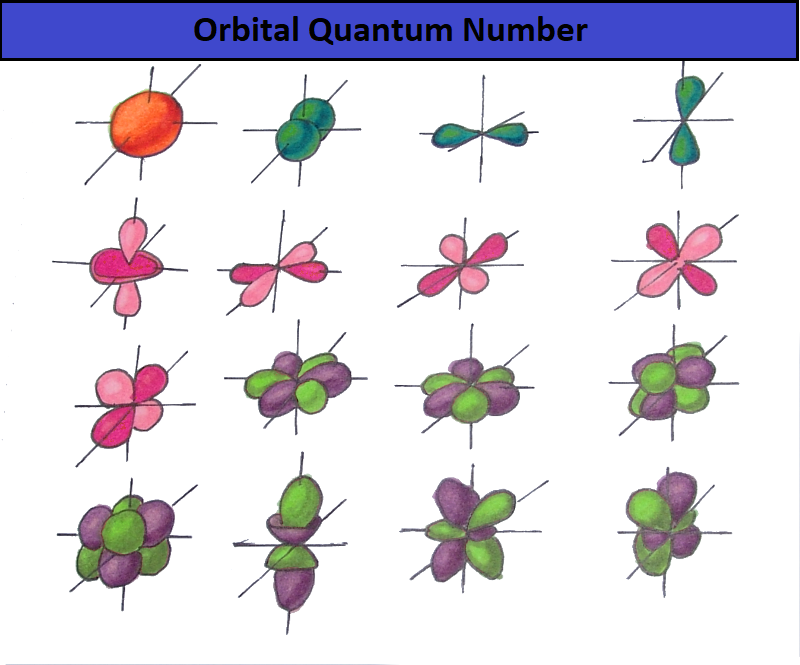To avoid confusion, use a simple scheme:

1. The s-shell has one orbital (L = 0) - two electrons.
2. The p-shell has three orbitals (L = 1) - six electrons.
3. The d-shell has five orbitals (L = 2) - ten electrons.
4. The f-shell has seven orbitals (L = 3) - fourteen electrons.

### How to Find Out the Valence and Fill in the Electronic Shell?

The rules for filling out electronic shells are as follows:

1. First, we find out how many electrons the atom of the element of interest to us contains. To do this, you enough to know the charge of its nucleus. It is always equal to the ordinal number of the element in the Periodic Table of Mendeleev. The serial number is exactly equal to the number of electrons in the entire atom.
2. Sequentially fill the orbitals, starting from the lower 1s-orbital, with the available electrons. In this case, it is impossible to place more than two electrons on each orbital.
3. We write down the electronic formula of the element. The electronic formula describes the distribution of electrons over the energy levels that exist in the electron cloud. This distribution is also called the electronic configuration of the atom. Having made the electronic configuration, you will know the element's valence.

User ratings:

User ratings is 4.3 stars.Henrique Bertulino